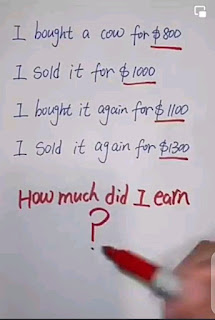0

# I Bought a cow for \$800 Math Quiz answerI Bought a cow for \$800 answer

The "I bought a for \$800 math Quiz" is one of those simple but tricky math Quiz on the net making people scratch their heads.

Today I will bring you the correct answer in detail in this post.

## What's the I Bought a cow for \$800 Math Quiz answer?

The correct answer would be \$400 not \$100 see details below.

Want more Puzzles and Quizzes?

Check here

I will solve in two cases

First case

I bought a cow 🐄 for \$800

I sold it for \$1000

Gain = Cost price-Selling Price

Cost price= \$800

Selling Price= \$1000

Gain = \$1000-\$800

Gain = \$200

Total Cash or Asset = \$1000

Second Case

Cost price = \$1100

Selling Price = 1300

Gain = 1300-1100

Gain = \$200

Gain of first case + Gain of Second cased
= \$200+ 200
= \$400

Therefore, the gain from the two business deal is \$400

However ever The Capital was just \$1000. To buy something for \$1100, You need to add \$100.

So, if You remove the \$100 you added, your Gain would be \$300.

Hence the final answer should be \$300

## Conclusion

To solve this type of Quiz, you need to go step by step else you will get the wrong answer

Also check: 10-10×10+10=? Math Quiz answer

Tags